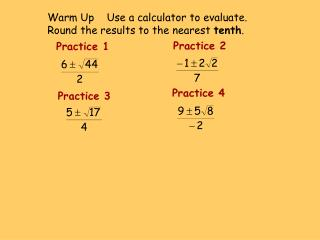Download PresentationPractice 1# Practice 1 - PowerPoint PPT Presentation

Download Presentation##### Practice 1

Download Policy: Content on the Website is provided to you AS IS for your information and personal use and may not be sold / licensed / shared on other websites without getting consent from its author. While downloading, if for some reason you are not able to download a presentation, the publisher may have deleted the file from their server.

- - - - - - - - - - - - - - - - - - - - - - - - - - - E N D - - - - - - - - - - - - - - - - - - - - - - - - - - -
##### Presentation Transcript

1. Warm Up Use a calculator to evaluate. Round the results to the nearest tenth. Practice 2 Practice 1 Practice 4 Practice 3

2. Warm Up Use a calculator to evaluate. Round the results to the nearest tenth. Practice 2 Practice 1 Practice 4 Practice 3

3. 9.4B Using the Discriminant Algebra 1 Glencoe McGraw-Hill Linda Stamper

4. The x-intercepts of the graph are the solutions, or roots, of the related equation Notice the first equation is equal to y and the second equation is equal to 0. Why is y = 0? Because at the x-intercept, y is equal to 0. function related equation

5. The solutions, or roots, are NOT ordered pairs. y y • • • • x x The solutions, or roots, are –4 and 1. The solutions, or roots, are –2 and 2.

6. Write the quadratic formula: What happens to the quadratic equation if the value under the radical is a zero? What is this the formula for?

7. If the vertex of the parabola is on the x-axis, there is one solution. It is the x-coordinate of the vertex. y • x There is one solution.

8. What do you predict will happen when there is a negative under the radical? What does the graph look like to support your answer?

9. If the parabola does not intersect the x-axis, then there is no real solution. y x There are no real solutions, or roots.

10. Another way to identify how many solutions there are to an equation, if any, is to use the discriminant. In the quadratic formula, the expression inside the radical is called the discriminant. discriminant b2 – 4ac The Number of Solutions of a Quadratic Equation If the discriminant is positive, then the equation has two solutions. If the discriminant is zero, then the equation has one solution. If the discriminant is negative, then the equation has noreal solution.

11. State the value of the discriminant. Then determine the number of real roots of the equation. Write problem. Identify values for a, b and c. Write discriminant expression. Substitute values for a, b, and c. (Place values in parentheses to avoid sign errors and change subtraction to addition.) Simplify to find the discriminant. Identify the number of solutions. two

12. State the value of the discriminant. Then determine the number of real roots of the equation. Example 3 Example 2 Example 1 one two no real roots

13. State the value of the discriminant. Then determine the number of real roots of the equation. Example 6 Example 5 Example 4 What is the related equation? two one no real roots

14. Homework 9-A13 Skills Practice Wkb. Page 60 #8-24.